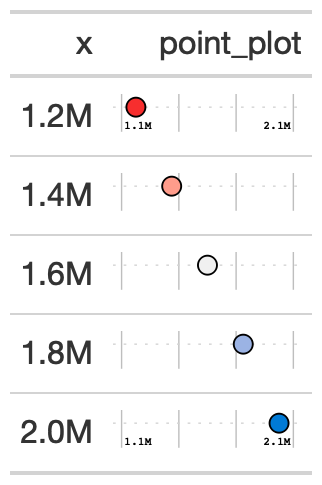Creates a dot/point plot in each row. Can be used as an alternative for a bar plot. Accepts any range of values, as opposed to gt_plt_percentile which is intended to be used for values between 0 and 100.

## Usage

gt_plt_point(
gt_object,
column,
width = 25,
scale = 1,
accuracy = 1
)

## Arguments

gt_object

An existing gt table

column

The column to transform to the percentile dot plot. Accepts tidyeval. All values must be end up being between 0 and 100.

palette

A vector of strings of length 3. Defaults to c('blue', 'lightgrey', 'red') as hex so c("#007ad6", "#f0f0f0", "#f72e2e")

width

A numeric, indicating the width of the plot in mm, defaults to 25

scale

A number to multiply/scale the values in the column by. Defaults to 1, but can also be 100 if you have decimals.

accuracy

Accuracy of the number labels in the plot, passed to scales::label_number()

a gt table

## Examples

point_tab <- dplyr::tibble(x = c(seq(1.2e6, 2e6, length.out = 5))) %>%
gt::gt() %>%
gt_duplicate_column(x,dupe_name = "point_plot") %>%
gt_plt_point(point_plot, accuracy = .1, width = 25) %>%
gt::fmt_number(x, suffixing = TRUE, decimals = 1)

## Figures## Function ID

3-9

Other Plotting: gt_plt_bar_pct(), gt_plt_bar_stack(), gt_plt_bar(), gt_plt_dist(), gt_plt_percentile(), gt_plt_sparkline(), gt_plt_winloss()